## Math 501: Differential Geometry

Homework 9 <me@tylerlogic.com>

April 26, 2013
http://coursework.tylerlogic.com/courses/math501/homework09

1

Because the parallel transport operation is a linear operation, then we can look at what happens to the two elements of the basis {(1,0,0),(0,1,0)} of the tangent space at the north pole. Because these two vectors span, it will inform us how arbitrary vectors in the space are changed by the parallel transport operation.

Let γ1, γ2, and γ3 be the three piece-wise curves that make up γ. Because each of the peice-wise curves are geodesics, then their derivative functions are parallel. Hence we have that the vector (1,0,0) will be transported to (0,0,-1) by γ1, transported from there to (0,0,-1) by γ2, and then transported to (0,1,0) by γ3, returning to the north pole. Similarly (0,1,0) is transported to (0,1,0) by γ1, then to (-1,0,0) by γ2, and finally to (-1,0,0) by γ3. Thus the overall transport of (1,0,0) and (0,1,0) along γ is to (0,1,0) and (-1,0,0), respectively. Since these two elements are a basis, the parallel transport is simply a rotation of the whole space by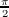radians.

2

(a)

Since S is a compact and orientable surface, its Euler characteristic, χ(S), is -2n + 2 for some natural number n. Thus if K > 0, the total curvature is positive, which in light of the Gauss-Bonet theorem for compact and orientable surfaces, SKdA = 2πχ(S), implies that χ(S) needs also to be positive, i.e. it is 2. Hence the compact orientable surface S is homeomorphic to a sphere, since it too is a compact orientable surface of Euler characteristic 2.

(b)

Let S be a compact orientable surface that is not homeomorphic to the sphere. Then its Euler characteristic is one of 0,-2,-4,, which, by the Gauss-Bonet theorem, implies that its total curvature is 0,-4π,-8π,.

Because S is compact, we know from the first problem of homework six that a plane brought in from far enough away until it first touches S, at some p, will be tangent to S. Thus there will be some neighborhood of p for which S will lie entirely on one side of the tangent plane, and because of that fact the curvature at p will be positive. The main point being that S has a point with positive curvature.

Finally, becuase there is a point of S with positive curvature and because its total curvature is zero or negative, then S must also contain a point of negative curvature. Furthermore, because the Gaussian curvature of a surface is continuous, its containing points of positive and negative curvature implies that it must also contain a point of zero curvature.

3

By the Gauss-Bonet theorem, we have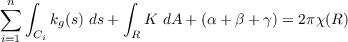but since the sides of R are geodesics, then each side has zero geodesic curvature. Also, because R is a subset of a sphere, χ(R) = 2 and K = 1. This leaves us with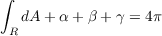from Gauss-Bonet theorem. Hence 4π - (α + β + γ) yields the area of R.

4 Total Curvature

(a)

From our midterm, we found isothermal coordinates for the catenoid rotated about the z-axis such that E = G = a2 cosh2t. For our current catenoid, a = 1. Thus E = G = cosh2t. From our eighth homework we developed a formula for Gaussian curvature for isothermal coordinates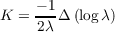where E = G = λ, which in our case boils down to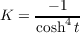This results in the following total curvature.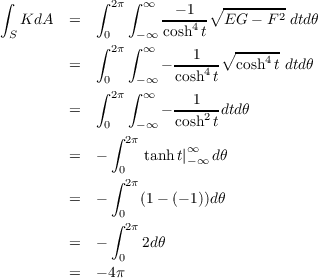(b)

Minimal surfaces have zero mean curvature at each point. This implies that at any point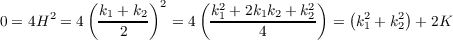where k1 and k2 are the principle curvatures at a point in S. Hence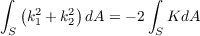or in other words the total curvature is finite whenever S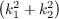dA is finite, and vice versa.

5

(a)

Let S be a surface homeomorphic to a sphere. From the second midterm we know that H2 K. Combining this with the Gauss-Bonet theorem, we get the following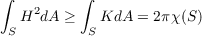which implies that SH2dA 4π, since the Euler characteristic of topological spheres, like S, is 2.

(b)

Let S be the sphere. Since the principle curvatures at a given point of the sphere are the same, then the sphere is an umbilical surface, i.e. k1 = k2 at each point. This indicates that k22 = k12 = K. Thus, using the Gauss-Bonet theorem for compact orientable surfaces like the sphere S, we get the following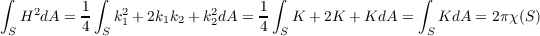which, for our case of the sphere, means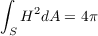since χ(S) = 2 for S.

Conversely, suppose that the Willmore energy of S is equal to 4π. Since S is a topological sphere, then χ(S) = 2, which implies, by the Gauss-Bonet theorem, that SKdA = 2πχ(S) = 4π. In other words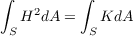Thus integrating over both sides of A.1, leaves us with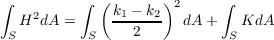implying that S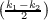2dA = 0. However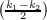2 is always non-negative, so because it’s integral is zero then it must be zero. This gives us the equality of the principle curvatures k1 and k2. Hence S must be a round sphere as a round sphere is the only topological sphere which is also umbilic.

6

Let S be a closed surface with some bounded subset B such that S \ B is contained within a plain. Also suppose that K 0 for all of S. Since B is bounded, we can “entriangle” it with a triangle contained in the same plane in which S \ B lies. Now define R to be the region of S containing this triangle and all of its interior (not to mention B). Because the boundary of R is a triangle that lies in a plane, then its edges are geodesics, and the sum of its interior angles is π. This implies that the sum of the geodesic curvature of its edges is zero and the sum of its exterior angles is 2π. Thus the Gauss-Bonet theorem informs us that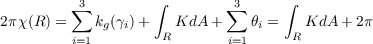(6.1)

where γi are the edges of R.

Now because R is bounded and closed (it contains the edges of the triangle), then it is compact due to Heine-Borel. Thus in light of the second hint in this problem’s statement R must have an Euler characteristic less than or equal to 1, boiling down section 6.1 to RKdA + 2π 2π or in other words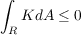However, because K 0, then the above integral can never be negative and must be zero, but this in turn implies that K = 0 since K was assumed to be non-negative.

### A Extra Lemmas

Lemma A.1 For any principles curvatures k1,k2 at some point of a regular surface, we that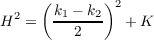Proof. The result comes from the following sequence of equations.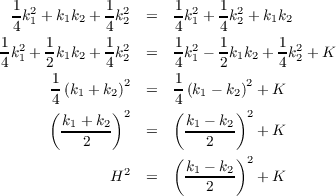__